# Test: Civil Engineering- 3

## 100 Questions MCQ Test Mock Test Series of SSC JE Civil Engineering | Test: Civil Engineering- 3

Description
Attempt Test: Civil Engineering- 3 | 100 questions in 60 minutes | Mock test for SSC preparation | Free important questions MCQ to study Mock Test Series of SSC JE Civil Engineering for SSC Exam | Download free PDF with solutions
QUESTION: 1

### Which one of the following metamorphic rocks has more weathering resistance characteristics?

Solution:

Quartzite is a hard, non-foliated metamorphic rock which was originally pure quartz sandstone. It has maximum weathering resistance characteristics among Limestone, phyllite, slate.

QUESTION: 2

### Rotary kiln used in manufacturing cement rotates at a speed of

Solution:

The burning process is carried out in the rotary kiln while the raw materials are rotated at 1-2 rpm at its longitudinal axis.

The rotary kiln is made up of steel tubes having the diameter of 2.5-3.0 meter and the length differs from 90-120 meter.

The inner side of the kiln is lined with refractory bricks.

QUESTION: 3

### When timber is burnt in the wood fire over a depth of about 15 mm, the process of treatment is known as______.

Solution:

Different methods of Timber Preservation are:

i) Brushing

ii) Spraying

iii) Injecting under pressure

iv) Dipping and stepping

v) Charring

vi) Hot and cold open tank treatment

Charring is basically a burning of timber surface (old method of preservation of timber). In this method, the timber surface is wetted for 30 minutes and burnt up to a depth of 15 mm from top surface. The burnt surface protects the inner timber from white ants, fungi, etc.

This method is not suitable for exterior wood works.

Dipping is another type of preserving the timber, In this method, timber is dipped directly in the preservative solution. Hence, the solution penetrates the timber better than the case of brushing or spraying.

In Hot and cold open tank treatment method, the timber is placed in an open tank which contains preservative solution. This solution is then heated for few hours at 85 to 95 degree Celsius. Then, the solution is allowed to cool, and timber gets submerged with this gradual cooling

QUESTION: 4

Which of the following compounds of Portland cement reacts immediately with the water and set early?

Solution:

Tricalcium Aluminate: C3A is formed within 24 hours of the addition of water in the cement and is responsible for maximum evolution of heat of hydration. It is the first compound that is formed after addition of water and sets early.

Tetracalcium aluminoferrite: C4AF is also formed within 24 hours of the addition of water in the cement but its individual contribution to the overall strength of the cement is insignificant.

Tricalcium silicate: C3S is formed within a week or so of the addition of water in the cement and is responsible for the early development of strength of the cement.

Dicalcium silicate: C2S is the last compound that is formed after the addition of water in the cement which may require a year or so for its formation. It is responsible for the progressive strength of the cement.

QUESTION: 5

Which one of the following is used as a carrier in paint?

Solution:

Carrier: It is an oily liquid in which the base and pigment are soluble. It facilitates the paint to be conveniently spread evenly over the surface by means of a brush. It acts as a binder for the base and cause it to stick to the surface.

Oils most commonly used as vehicles are: Linseed oil, Poppy oil. Nut oil and Tung oil.

Driers: These are metallic compounds which when added to the paint in small quantities accelerate the process of drying of the paint. Driers have a tendency to affect the colour of the paint and also to destroy the elasticity of the paint.

Litharge, manganese dioxide, lead acetate and cobalt are the usual driers, out of which litharge is most commonly used.

QUESTION: 6

I.S Code specifies that the compressive strength of brick shall be determined by keeping the brick:

i) Place the specimen with flat faces horizontal

ii) Place the specimen with flat faces vertical

iii) After soaking in water for 12 hrs

Solution:

As per IS 3495 (part 1): 1992, Procedure for testing of the compressive strength of Burnt clay solid brick is:

1. Place the specimen with flat faces horizontal, and mortar filled face facing upwards between two three-ply plywood sheets each of 3 mm thickness and carefully centered between plates of the testing machine.

2. Apply load axially at a uniform rate of 14 N/mm* (140 kgf/cm2) per minute till failure occurs and note the maximum load at failure.

3. The load at failure shall be the maximum load at which the specimen fails to produce any further increase in the indicator reading on the testing machine.

Preconditioning before the test:

Immerse of brick in water at room temperature for 24 hours.

QUESTION: 7

What is the recommended moisture content of timber, which is used as a structural element for windows?

Solution:

As per IS 287:1993:

The recommended moisture content of timber used as a structural element for windows is depend upon Zone area and thickness.

It lies between 10 to 16% for thickness of 50 mm and above

It lies between 8 to 14% for thickness less than 50 mm.

QUESTION: 8

The opening left in flat roofs for lightening purposes is called:

Solution:

The opening left in flat roofs for lightening purposes is known as Lantern.

Dormer windows are provided for sloped roofs. They provide ventilation as well as lighting to the room. They also enhance aesthetic sense of room.

Skylights are generally provided on the top of sloped roofs. To admit light into the rooms, sky lights are provided. It is provided parallel to the sloping surface. Sky lights can be opened when we required.

QUESTION: 9

The compressive strength of high duty bricks should be more than:

Solution:

As per IS 2180 : 1988:

Heavy duty burnt clay bricks shall be manufactured either by pressing or extrusion. They shall be made from a processed clay or clays blended in right proportion.

Average compressive strength of heavy-duty bricks shall not be less than 40 N/mm2

QUESTION: 10

Pallet board is used:

Solution:

The essential tools used in the hand moulding process are:

i) a brick mould

ii) cutting wire or strike

iii) wooden plates called pallets

iv) a stock board

Frog mark of bricks are made by using a pair of pallet boards.

QUESTION: 11

The hydraulicity of the hydraulic lime is mainly due to _____.

Solution:

Clay imparts hydraulicity to the lime and makes it insoluble in water, if it is in excess it arrests slaking if it is in deficiency it retards slaking.

Presence of sulphur in small quantity reduces the slaking and accelerates the setting process of lime.

QUESTION: 12

In plastering, the 1st coat is called ___________ and its thickness should be _______ mm.

Solution:

The first coat applied to the newly wall is called as “undercoat”.

In case of brick masonry, the thickness of first coat plaster is in general 12 mm and in case of concrete masonry this thickness varies from 9 to 15 mm.

QUESTION: 13

A diesel generator has been installed in a building at a cost of Rs. 3500.00. The amount of annual instalment of the sinking fund required to be deposited to accumulate the whole amount at 5 % rate of interest will be?

Assume life of the generator as 20 years

Solution:

Annual sinking fund (I) is given by: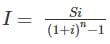Where,

S = total amount of sinking fund to be accumulated = 3500 Rs

i = rate of interest = 5% = 0.05

n = number of years required to be accumulate the sinking fund = 20 years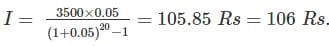QUESTION: 14

The cost of brickwork required in a wall of 5 m long, 3.5 m high and 20 cm thick if the rate of brick work is 345 per cubic meter is?

Solution:

Quantity of brickwork = L x B x H

= 5 x 3.5 x 0.20

= 3.5 m3

Cost of 1 m3 of brickwork = Rs. 345

Cost of 3.5 m3 of brickwork = 3.5 x 345 = Rs. 1207.5

QUESTION: 15

When one engineering department takes up the work of other department, the Centage charges are taken to be:

Solution:

Centage charges: When the engineering department takes up the work of other department, a percentage amount of 10 to 15 % of the estimate cost is charged to meet the expenses of establishment, designing, planning, supervision etc and this percentage charge is known as Centage charge.

QUESTION: 16

Which among the following item is not included in technical sanction of the work?

Solution:

Technical sanction means the sanction of the detailed estimate, design calculations, quantities of work, rates and cost of the work by the competent authority of the engineering department. After the technical sanction of the estimate is given then only the work is taken up for construction.

Note: Fund allotment is a part of Expenditure sanction.

QUESTION: 17

The expected out turn of the cement concrete 1 : 2 : 4 for per mason per day will be:

Solution:

The capacity of doing work by an artesian or skilled labour in the form of quantity of work per day is known as out turn of the labour.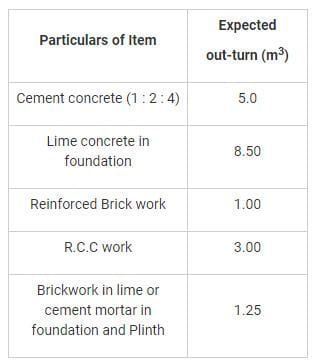QUESTION: 18

Chowkhats are measured in:

Solution:

i) Doors and window frames or chowkhats are computed in cubic meter.

ii) Door or Window Leaves or Shutter are computed in square meter.

iii) Wood work such as wooden beams, burgahs, posts, wooden roof trusses, chowkhats etc are computed in cubic meter.

QUESTION: 19

Which among the following is not included in the Circulation area of the building?

Solution:

Circulation area is the floor area of verandahs, passages, corridors, balconies, entrance hall, porches, stair cases etc. which are used for movements of persons using the building.

The Circulation area shall comprise of the following items:

i) Verandahs and balconies

ii) Passages and corridors

iii) Entrance halls

iv) Stair case and mumties

v) Shafts for lifts

QUESTION: 20

Which among the following item is not included in tender notice for a particular project?

Solution:

Items included in Tender notice are:

i) Description of work

ii) Estimated cost of work

iii) Date and Time of the tender

iv) Amount of Earnest money

v) Security money

vi) Time of completion

QUESTION: 21

Skirting up to 30 cm in height shall be measured in:

Solution:

Skirting up to 30 cm in height shall be measured in running meter while skirting or dado exceeding 30 cm shall be measured in square meter stating the type of finish.

QUESTION: 22

What is the degree of the curve (in degree) for a radius of 573 m using chain of 20 m length?

Solution:

For 20 m chain length,

Degree of curve (D) is given by: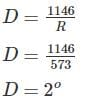Note:

For 30 m chain length,

Degree of curve (D) is given by: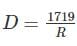QUESTION: 23

The length of a line measured with chain having 100 links was found to be 2000 links. If the chain was 0.5 links too short, find the true length of the line.

Solution:

Let True length of the line = L

True or designated length of chain (l) = 100 links

Wrong length of line measured (L’) = 2000 links

Wrong length of the chain (l’) = 100 - 0.5 = 99.5 links

l’ × L’ = l × L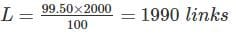QUESTION: 24

The hypotenuse allowance (in m) for 30 m long chain, if the slope is 1:10 is __________.

Solution:

Hypotenuse allowance (Cm) for 30 m chain is given by: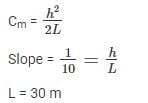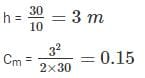QUESTION: 25

Radius of visible horizon from top of a 134 m light house will be:

Solution:

Distance of visible horizon is given by: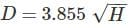H = height of visible horizon (meters)

D = Distance of visible horizon (km)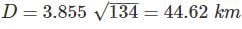QUESTION: 26

How much refraction correction (in m) is required for a distance of 500 m?

Solution:

Refraction correction is given by: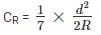Where,

d = distance in (meters)

R = radius of earth = 6370 km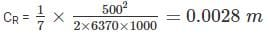QUESTION: 27

A sample of soil has liquid limit 50%, plastic limit 25%, shrinkage limit 20% and moisture content 35%. The consistency index is _________

Solution:

Liquid limit, WL = 50%

Plastic limit, WP = 25%

Moisture content, W = 35%

Consistency index,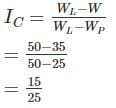QUESTION: 28

The ratio of the difference between the void ratio of the soil in its loosest state and its natural void ratio (e) to the difference between the void ratios in the loosest and fully dense state, is generally termed as _____.

Solution:

Void ratio in loosest state = emax

Void ratio in dense state = emin

Natural void ratio = e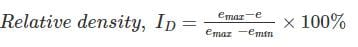So the ratio of the difference between the void ratio of soil in loosest state and its natural void ratio to the difference between the void ratio in loosest state and fully dense state is called Relative density.

By relative density, the field compaction is how much achieved is determined for coarse grained soil.

QUESTION: 29

The Relative density of compacted dense sand is approximately equal to ________.

Solution:

The relative density of compacted dense sand is experimentally observed to be 0.95.

QUESTION: 30

The soil mass under consideration represents _______ when the degree of saturation is zero

Solution:
QUESTION: 31

The consolidation settlement is given by the expression -

Solution:

The consolidation settlement is given by,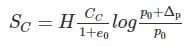Where CC = Co-efficient of the compression index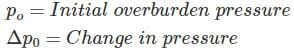QUESTION: 32

If voids ratio is 0.67, water content is 0.188 and specific gravity is 2.68, the degree of saturation of the soil, is _____.

Solution:

Void ratio, e = 0.67

Water content, w = 0.188

Specific gravity, G = 2.68

We know, eSr = wG

Where S= Degree of saturation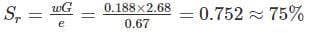QUESTION: 33

A moist sand occupies a depth of 15 cm and when fully saturated it occupies 12 cm, Then the bulking of the moist sand is

Solution: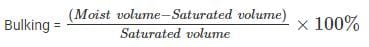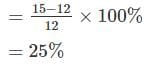QUESTION: 34

In the case of cohesive soil, active earth pressure at the top is ______

(where C = Cohesion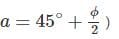Solution:

In case of cohesive soil, active earth pressure at top is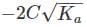Where Ka = Co-efficient of active earth pressure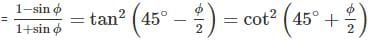So intensity of active earth pressure at top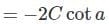QUESTION: 35

The apparatus that is suitable for conducting drained, undrained and consolidated drained test is-

Solution:
QUESTION: 36

For a smooth hump in a subcritical flow to function as a broad crested weir, the height ΔZ of the hump above the bed must satisfy which one of the following?

(E1 = Specific head upstream of the hump.

EC = Specific head at the critical depth yc)

(Neglect friction effects)

Solution:

If the flow is subcritical, then after the introduction of hump the specific energy will decrease by ΔZ (height of hump).

Ec ≤ E1 – ΔZ

⇒ ΔZ ≤ E1 – Ec

QUESTION: 37

Specific speed of a Pelton turbine ranges from

Solution:

Specific speed: It is defined as the speed of a similar turbine working under a head of 1 m to produce a power output of 1 kW. The specific speed is useful to compare the performance of various type of turbines. The specific speed differs for different type of turbines and is same for the model and actual turbine.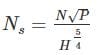1. The specific speed of Pelton wheel turbine (single jet) is in the range of 10-35

2. The specific speed of Pelton wheel turbine (multiple jets) is in the range of 35-60

3. The specific speed of Francis turbine is in the range of 60-300.

4. The specific speed of Kaplan turbine is greater than 300.

QUESTION: 38

The sequent-depth ratio in a hydraulic jump formed in a horizontal rectangular channel is 2. The Froude number of the supercritical stream is

Solution:

Using the relation: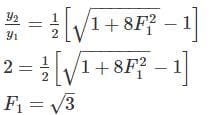Where,

y2 = Post jump depth

y1 = Pre-jump depth

F1 = Froude number before the jump

QUESTION: 39

The pressure _________ as the depth of the liquid increases

Solution:
QUESTION: 40

Which of the following advantages is/are possessed by a Kaplan turbine over a Francis turbine?

1. Low frictional losses

2. Part load efficiency is considerably high

3. More compact and smaller in size

Select the correct answer using the codes given below.

Solution:
• In Francis turbine the contact surface b/w solid and liquid is more due to more no. of blades hence losses in runner is high. In Kaplan turbine the contact surface b/w solid and liquid is less due to less no. of blades so losses in runner is less
• In Francis Turbine mounting of shaft may be vertical or horizontal in direction. In Kaplan Turbine mounting of shaft is only in vertical direction
• In Francis Turbine Runner vanes are fixed with the shaft, so it can’t be adjusted. In Kaplan Turbine Runner vanes are adjustable
• In Francis Turbine the medium head is required generally in the range of (100-500) meter. In Kaplan Turbine very low head is required, generally less than 100-meter
• In Francis Turbine medium flow rate is required. In Kaplan Turbine very large flow rate is required
• Part load efficiency is considerably higher for Kaplan turbine
QUESTION: 41

Match List I with List II and select the correct answer using the codes given below the lists.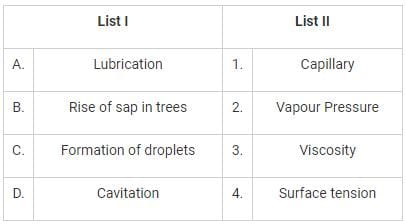Solution:

From the given table provided in question, we can conclude that list 2 is a property and list 1 is application of that property.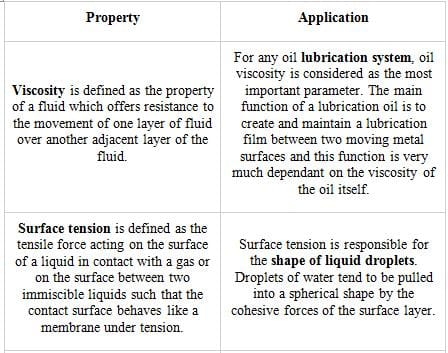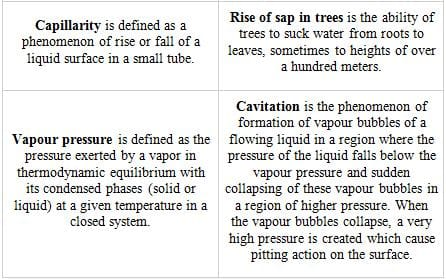QUESTION: 42

Chezy’s equation is used to determine

Solution:

The Chezy equation can be used to calculate mean flow velocity in open channel.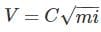• C is Chezy's coefficient
• R is the hydraulic radius (Area/Perimeter)
• I is loss of head per unit length of pipe (hf/L)
QUESTION: 43

A two – dimensional flow is described by velocity components u = 2x and v = - 2y. The discharge between points (1, 1) and (2, 2) is equal to

Solution: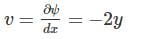………(i)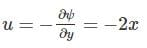………(ii)

Integrating (i), we get

ψ = – 2xy + f(y)                                 ………(iii)

Differentiating (iii) w.r.t. y. We get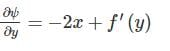………(iv)

Equating (ii) and (iv), we get

f’(y) = 0

Integrating (v), we get

f(y) = C

where C is a numerical constant which can be treated as zero

From (iii), we get

ψ = - 2 xy

At (1, 1); ψ1 = -2 units

At (2, 2); ψ2 = - 8 units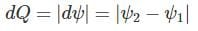= 8 – 2

= 6 units

QUESTION: 44

Uniform flow in a channel is characterized by the following statements:

Solution:

The depth, slope and velocity remain constant along the channel. The water surface slope, the slope of energy line and the bed slope will all be equal to each other.

QUESTION: 45

On rolling land, the method of applying water is:

Solution:

In free flooding, Ditches are excavated in the field either on the contour or up and down the slope. This method is suitable for close growing crops, pastures etc. This method is suitable on rolling land of irregular topography, where other methods are not suitable.

In Border flooding, the land is divided in to number of strips, separated by low levees called borders.

Check flooding is similar to ordinary flooding except that the water is controlled by surrounding the check area with low and flat levees. This method is suitable for more permeable soils as well as for less permeable soils.

In furrow flooding method, only one-fifth to one-half of the land surface is wetted by water. In this method, evaporation loss is very low

QUESTION: 46

Blaney-Criddle method is used to determine:

Solution:

The Blaney-Criddle method is used to determine evapotranspiration i.e. consumptive use of the crop.

It is one of the oldest methods to measure evapotranspiration (Consumptive use of water).

QUESTION: 47

Estimation of peak flood discharge for a catchment measuring 180 sq.km is done using Dicken’s formula with a coeff C = 16. The peak flood discharge would be:

Solution:

Catchment area A = 180 km2

Design Discharge,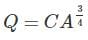A = Catchment area (km2)

C = 16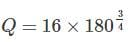Q = 786.27 m3/s

QUESTION: 48

Consider the following statements: An aqueduct is a cross drainage work in which

1. a canal is carried over the drainage channel

2. a drainage channel is carried over the canal

3. both drainage channel and canal are at the same level

Which of these statements is/are correct?

Solution:

An aqueduct and syphon aqueduct are cross drainage works in which the canal is taken over the natural drain, such that the drainage water runs below the canal either freely or under syphoning pressure.

Super passage and syphon are cross drainage works in which the drain is taken over the canal such that the canal water runs below the drain either freely or under syphoning pressure.

The level crossing is a cross drainage work in which the canal water and drain water are allowed to intermingle with each other. A level crossing is generally provided when a large canal and a huge drainage (such as a stream or a river) approach each other practically at the same level.

QUESTION: 49

Consumptive use coefficient for crops like wheat, barley, flax, and other small grains is approximately:

Solution:

The ratio of Evapotranspiration (Consumptive use) to the Pan evaporation is known as consumptive use coefficient.

It is different for different crops and is different for same crops at different places.

The different crops are divided in to 8 groups:

The crops like wheat, barley, flax, and other small grains is lies in Group D and the maximum value of Consumptive use coefficient for these crops is 0.90.

For crops like melons, onions carrots and grapes lies in Group C, the maximum value of Consumptive use coefficient for these crops is 0.60.

QUESTION: 50

Turbidity of a water sample depends on

Solution:

Turbidity of water sample depends on the concentration and density of suspended and colloidal solids.

Turbidity doesn’t depend upon the dissolved solids as the dissolved solids remain in ionic form.

QUESTION: 51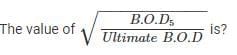Solution:

BODis given by: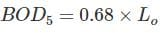Where,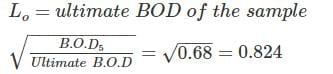QUESTION: 52

Water requirement per day per bed in 250 beds hospital according to IS 1172:1992 is?

Solution:

As per IS 1172:1993, water requirement for buildings other than residences are: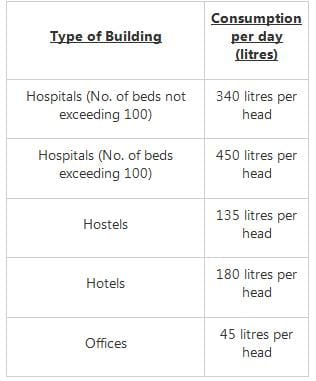QUESTION: 53

Which one is not the effect of the presence of iron oxide in water?

Solution:

Iron is found on earth mainly as insoluble ferric oxide. Iron can impart bad taste to the water, causes discolouration in clothes and incrustations in water mains.

i) As it contains multivalent cations which is responsible for hardness in water.

ii) Reaction of iron oxide with water will lead to increase in concentration of CO2 which is responsible for the corrosiveness in water.

iii) As colour of iron oxide is red, so it induces red colour in water.

iv) Presence of iron oxide does not cause toxic effect in water.

QUESTION: 54

Electrostatic precipitators are used as pollution control device for the separation of:

Solution:

The electrostatic precipitator is one of the most widely used collection devices for particulates. An electrostatic precipitator (ESP) is a particulate collection device that removes particles from a flowing gaseous stream (such as air) using the force of an induced electrostatic charge.

ESP can be operated at high temperature and pressures, and its power requirement is low. An electrostatic precipitator is a type of filter (dry scrubber) that uses static electricity to remove soot and ash from exhaust fumes before they exit the smokestacks.

QUESTION: 55

The shape of the camber best suited for cement concrete pavements is:

Solution:

Camber is the slope provided in the transverse direction of the road to drain off the rain water from the road surface. Usually camber is provided in the straight roads by raising the centre of the carriage way with respect to the edges forming highest point at the centre.

Straight slope of camber is easier to be cast and maintained in case of concrete pavement. Parabolic shaped camber is difficult to construct and maintain. It has more steeper edges which are inconvenient to use. However, it is kept in bituminous pavements for better drainage of water.

QUESTION: 56

The width of carriageway for multi-lane pavements as recommended by Indian roads congress is:

Solution:

Width of carriageway for the type of lane as recommended by IRC is: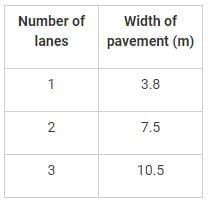QUESTION: 57

For a comfortable travel on highways, the centrifugal ratio should not exceed:

Solution:

Centrifugal ratio is given by: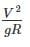V = design speed

For highways, Centrifugal ratio = ¼ = 0.25

For Railways, Centrifugal ratio = 1/8 = 0.125

QUESTION: 58

As per IRC, the minimum elastic recovery for crumb rubber modified bitumen should be:

Solution:

According to IRC,

The minimum elastic recovery for crumb rubber modified bitumen should be 50%.

QUESTION: 59

Steel structures are ideally suitable for impact loads because they have high:

Solution:

Toughness is defined as the ability of a metal to resist fracture. It is significant for ductile materials only.

Mild steel is a ductile material having high fracture strain, fracture in the material occurs under impact loads and the material which have high fracture strain have high value of toughness.

QUESTION: 60

When the load line coincides with the centroid of the rivet group, the rivets are subjected to:

Solution:
QUESTION: 61

At any point of a beam, the section modulus may be obtained by dividing the moment of inertia of the section by _____.

Solution:
QUESTION: 62

Kelly ball apparatus is used for:

Solution:

Learn the following statements:

i) Slump test, compaction factor test, Kelly ball apparatus, vee-bee consistometer is used for measurement of workability.

ii) Vicat's apparatus is used to measure consistency of cement.

iii) Universal testing machine is used for measurement of strength.

QUESTION: 63

Consider the following statements:

Entrainment of air in concrete is done so as to

1. Increase the workability

2. Increase the strength

3. Increase the resistance to freezing and thawing

Which of these statements is/are correct?

Solution:

Air entrained concrete has effects on the compressive strength of concrete and its workability. Air entrained concrete increases the workability of concrete without much increase in the water-cement ratio.

To maintain the desired compressive strength and workability of concrete together, generally, in the case of higher strength concrete, admixtures are used. Air entraining agent is one such concrete admixture to increase the workability without affecting much reduction in compressive strength.

QUESTION: 64

Consolidation of concrete can be improved:

Solution:

Consolidation is the process of inducing a closer arrangement of the solid particles in freshly mixed concrete or mortar during placement by the reduction of voids, usually by vibration, centrifugation, rodding, tamping, or some combination of these actions. It can be improved before placing by any of the above means.

QUESTION: 65

Which of the following has lowest heat of hydration?

Solution:

Heat of hydration for C2S is lowest among all compounds.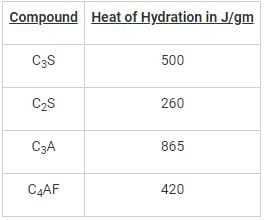QUESTION: 66

Which one of the following is correct regarding the most effective requirements of durability in concrete?

Solution:

Lower water cement ratio results in higher strength and durability of cement due to reduced voids. Type of cement is also responsible for engineering behaviour of concrete.

QUESTION: 67

The result of Vee-Bee test is expressed in terms of:

Solution:

Vee-Bee consistometer test is used for the concrete which possess low workability, slump for which is limited to 50 mm.

The measurement of the effort is done by time measurement in seconds. The amount of work measured in seconds is called as the remoulding effort. The time required for the complete remoulding is a measure of the workability and is expressed in the Vee-Bee seconds.

QUESTION: 68

Consider the following statements:

When cement is tested for setting time; on gauging its shows quick setting. This phenomenon known as “Flash set” of cement is due to the presence of high

1. Tricalcium Aluminate (C3A) in cement.

2. Tricalcium silicate (C3S) in cement.

Which of these statements are correct?

Solution:

Tri-calcium aluminate is reacts very fast with water and may lead to immediate stiffening of the paste. This process is known as flash set. Gypsum is added to cement to prevent such a fast reaction.

QUESTION: 69

Which one if the following is employed to determine strength of hardened existing concrete structure?

Solution:

Rebound hammer and ultrasonic pulse velocity are the two methods for assessing properties of hardened concrete.

Both the test are Non-destructive test.

QUESTION: 70

Which of the following roles fly ash does not play in concrete?

Solution:

The use of fly ash in portland cement concrete (PCC) has many benefits and improves concrete performance in both the fresh and hardened state. Fly ash use in concrete improves the workability of plastic concrete, and the strength and durability of hardened concrete. Fly ash use is also cost effective. When fly ash is added to concrete, the amount of portland cement may be reduced

Benefits of fly ash in Fresh Concrete:

Improved workability: The spherical shaped particles of fly ash act as miniature ball bearings within the concrete mix, thus providing a lubricant effect i.e. improved in workability is seen.

Reduced heat of hydration: Replacing cement with the same amount of fly ash can reduce the heat of hydration of concrete.

Decreased water demand: The replacement of cement by fly ash reduces the water demand for a given slump. When fly ash is used at about 20 percent of the total cementitious, water demand is reduced by approximately 10 percent.

Benefits of fly ash in Hardened Concrete:

Increased ultimate strength:  The additional binder produced by the fly ash reaction with available lime allows fly ash concrete to continue to gain strength over time.

Reduced permeability: The decrease in water content combined with the production of additional cementitious compounds reduces the pore interconnectivity of concrete, thus decreasing permeability.

Improved durability:  The decrease in free lime and the resulting increase in cementitious compounds, combined with the reduction in permeability enhance concrete durability.

Note: Addition of fly ash in concrete will lead to decrease in concrete available to hydration, thus it deaccelerates the strength gain of concrete.

QUESTION: 71

Identify the wrong statement:

Solution:

Bulking of sand: The increase in the volume of sand due to increase in moisture content is known as bulking of sand. A film of water is created around the sand particles which forces the particles to get a side from each other and thus the volume is increased.

Bulking of sand is maximum at 4.6 % moisture content.

Five to eight percent of the increase in moisture in sand can increase the volume of sand up to 20 to 40 percent.

The measurement of materials for making concrete is known as batching. Generally batching of concrete is done by two methods:

i) Volume batching

ii) Weight batching

Volume batching is not ideal method of batching. Wet sand has higher volume for the same weight of dry sand. It is called bulking of sand. Hence it upsets the calculated volume required. So that’s why it is important to consider the bulking effect of sand while volume batching.

QUESTION: 72

How many components are mainly used to prepare concrete?

Solution:

Four components are mainly used to prepare concrete:

i) cement

ii) Sand

iii) aggregates

iv) water

QUESTION: 73

What is the correct sequence of operations involved in concrete production?

Solution:

Batching is preferably done by weigh method and correct sequence of operations involved in concrete production are:

i) Batching

ii) Mixing

iii) Handling

iv) Transportation

Note:Batching is the process, in which the quantity or proportion of materials like cement, aggregates, water, etc. are measured on the basis of weight or volume to prepare the concrete mix

QUESTION: 74

The risk of segregation is more for:-

Solution:

In wetter mix as the water content is more chances of segregation is more. If there is large proportion of maximum size aggregate or coarse grading, segregation may occur.

QUESTION: 75

The aggregate is said to be elongated if its length is:

Solution:

The aggregate is said to be elongated if its greater length is greater than 9/5th of its mean dimension.

Elongated aggregates are unsuitable for pavement design.

Elongated aggregates can be found with the help of length gauge.

QUESTION: 76

Fineness modulus is:

Solution:

Fineness modulus of aggregates represents the average size of the particles in the aggregate by an index number. It is calculated by performing sieve analysis with standard sieves. Fineness modulus is generally used to get an idea of how coarse or fine the aggregate is.

More fineness modulus value indicates that the aggregate is coarser and small value of fineness modulus indicates that the aggregate is finer.

QUESTION: 77

The resistance of an aggregate to wear is known as:

Solution:

Abrasion test is carried out to test the hardness property of aggregates and to decide whether they are suitable for different pavement construction works. Los Angeles abrasion test is a preferred one for carrying out the hardness property and has been standardized in India (IS:2386 part-IV). The principle of Los Angeles abrasion test is to find the percentage wear due to relative rubbing action between the aggregate and steel balls used as the abrasive charge.

QUESTION: 78

What is the percentage of the fine aggregate of fineness modulus 2.6 to be combined with coarse aggregate of fineness modulus 6.8 for obtaining combined aggregate of fineness modulus 5.4?

Solution:

The percentage of fine aggregate is given by: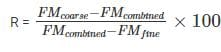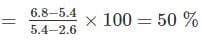QUESTION: 79

As per IS 456:1978, the ratio of stress in concrete to its characteristics strength at collapse in flexure for design purposes is taken as:

Solution:

According to IS 456:1978,

The compressive strength of concrete in the structures shall be assumed to be 0.67 times the characteristic strength. The partial safety factor of 1.5 shall be applied in addition to this.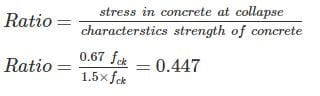QUESTION: 80

The reason for choosing mild steel bars as reinforcement in tensile zones of concrete is that

Solution:

The reason for choosing mild steel bars as reinforcement in tensile zones of concrete is that the coefficient of linear expansion is about the same as that of concrete as strain in concrete and steel should be same for perfect bond.

QUESTION: 81

Design of a two-way slab simply supported on edges and having no provision to prevent the corners from lifting, is made by _____.

Solution:

Design of a two-way slab simply supported on edges and having no provision to prevent the corners from lifting, is made by Rankine Grashoff formula.

QUESTION: 82

What is the value of minimum reinforcement (in case of mild bars) in a slab?

Solution: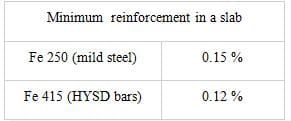QUESTION: 83

Choose the correct option with reference to IS 456:2000, for singly reinforced section:

Solution:
QUESTION: 84

The load on a lintel is assumed as uniformly distributed, if the masonry above it is up to a height of:

Solution:

A lintel is a horizontal member which is placed across the openings like doors, windows etc. It takes the load coming from the superstructure above it and gives support.

While designing the lintels, it is assumed that the load on the lintel is uniformly distributed if the masonry above it is up to a height of 1.25 times the effective span.

QUESTION: 85

If nominal shear stress is less than design shear strength of concrete, then:

Solution:

The minimum shear reinforcement is provided for the following:

(i) Any sudden failure of beams is prevented if concrete cover bursts and the bond to the tension steel is lost.

(ii) Brittle shear failure is arrested which would have occurred without shear reinforcement.

(iii) Tension failure is prevented which would have occurred due to shrinkage, thermal stresses and internal cracking in beams.

(iv) To hold the reinforcement in place when concrete is poured.

(v) The section becomes effective with the tie effect of the compression steel.

QUESTION: 86

A cantilever beam has span of 3.5 m. The effective depth of beam as per deflection criteria should be:

Solution:

For the deflection requirements:

Different basic values of span to effective depth ratios for three different support conditions are prescribed for spans up to 10 m, which should be modified under any or all of the four different situations: (i) for spans above 10 m, (ii) depending on the amount and the stress of tension steel reinforcement, (iii) depending on the amount of compression reinforcement, and (iv) for flanged beams.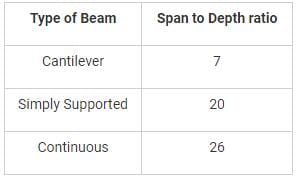So, in this case: span/effective depth (d) = 7

d= 3500/7= 500 mm

QUESTION: 87

At a point in a stressed material, normal stresses are 120 N/mm2 in x-direction and 80 N/mm2 in y-direction. The normal stres on a plane at 30° to the plane of 120 N/mm2 is-

Solution:
QUESTION: 88

Young’s modulus of elasticity is defined as the ratio of which of the following?

Solution:

Young's Modulus of Rigidity is the ratio of longitudinal stress to longitudinal strain.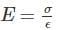Shear Modulus or Modulus of rigidity is the shear stress to shear strain.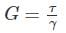Possion's ratio is the ratio of lateral strain to longitudinal stress.

Bulk Modulus of elasticity is the ratio of volumetric stress to volumetric strain.

QUESTION: 89

If a simply supported beam AB of the span L is subjected to an external moment M at distance a from end A, the reactions RA and RB are-

Solution:
QUESTION: 90

If a body is transmitting torque T N - m at N rpm, then power (in Watts) transmitted will be:-

Solution:

Power is defined as the rate of work done.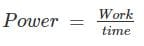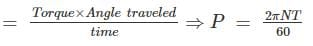QUESTION: 91

A is a shaft of diameter d and B is a shaft of a diameter 2d. The ratio of polar modulus of section B to section A is _____.

Solution:

Polar modulus,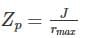Where J = polar moment of inertia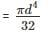Z is polar section modulus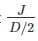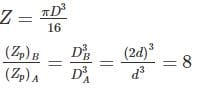QUESTION: 92

A propped cantilever of span L is fixed at end A and simply supported at end B. Due to a concentrated load W at midspan reaction at B is -

Solution:
QUESTION: 93

What is the deflection at the hinge for the beam shown below? (EI = constant)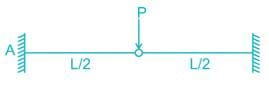Solution:
QUESTION: 94

The limiting slenderness ratio for applying Euler’s theory for columns with Young’s modulus E and crushing strength fc is

Solution:

According to Euler’s theory, critical load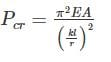Where, A = Cross-sectional area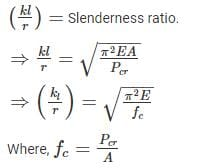QUESTION: 95

A parabolic arch has spring hinge A and B are at different levels. The height of crown point C from A is h1 and from B it is h2. If L is a span, the horizontal distance of AC is -

Solution:
QUESTION: 96

In a general two-dimensionally stressed elemets the normal stresses are px = 120 N/mm2, py = 80 N/mm2 and shear stress is 15 N/mm2. The maximum shear stress is _____

Solution:

The maximum shear stress,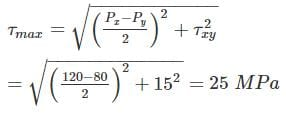QUESTION: 97

A bar of brass is enclosed in a steel tube and are rigidly fastened at both the ends. If the coefficient of thermal expansion of brass is more than that of the steel, when the temperature rises, the nature of stresses developed are-

Solution:
QUESTION: 98

A circular arch of span 25 m with a central rise 5 m is hinged at the crown and springings. It carries a point load of 100 kN at 5 m from the left support. In this case, the horizontal thrust is

Solution:
QUESTION: 99

What is the Distribution factor of member BA in the portal frame given below?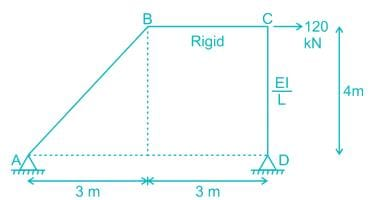Solution: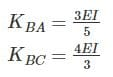Distribution factor of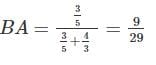QUESTION: 100

What is the degree of kinematic indeterminacy for the beam shown below (links have no loading on it)?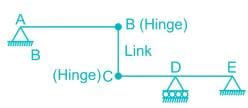Solution:

Degree of Kinematic indeterminacy is given by:

Dk = 3j - r+ r- nr

Where,

j = No. of Joints

re = External support reactions

rr = Released reactions

= 3 x 5 – 5 + 2 – 4 = 8Use Code STAYHOME200 and get INR 200 additional OFF Use Coupon Code